Practice Test: Circles

# Practice Test: Circles

Test Description

## 25 Questions MCQ Test NCERT Mathematics for CAT Preparation | Practice Test: Circles

Practice Test: Circles for Class 10 2022 is part of NCERT Mathematics for CAT Preparation preparation. The Practice Test: Circles questions and answers have been prepared according to the Class 10 exam syllabus.The Practice Test: Circles MCQs are made for Class 10 2022 Exam. Find important definitions, questions, notes, meanings, examples, exercises, MCQs and online tests for Practice Test: Circles below.
Solutions of Practice Test: Circles questions in English are available as part of our NCERT Mathematics for CAT Preparation for Class 10 & Practice Test: Circles solutions in Hindi for NCERT Mathematics for CAT Preparation course. Download more important topics, notes, lectures and mock test series for Class 10 Exam by signing up for free. Attempt Practice Test: Circles | 25 questions in 25 minutes | Mock test for Class 10 preparation | Free important questions MCQ to study NCERT Mathematics for CAT Preparation for Class 10 Exam | Download free PDF with solutions
 1 Crore+ students have signed up on EduRev. Have you?
Practice Test: Circles - Question 1

### A point P is 10 cm from the centre of a circle. The length of the tangent drawn from P to the circle is 8 cm. The radius of the circle is equal to

Detailed Solution for Practice Test: Circles - Question 1

ΔPTO is a right angled triangle at T ,where the tangent touches the circle.
So applying pythagoras theorem,
H2=P2+B2
102=B2+82
B=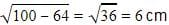Practice Test: Circles - Question 2

### A point P is 25 cm from the centre of a circle. The radius of the circle is 7 cm and length of the tangent drawn from P to the circle is x cm. The value of x =

Practice Test: Circles - Question 3

### In fig, O is the centre of the circle, CA is tangent at A and CB is tangent at B drawn to the circle. If ∠ACB = 75°, then ∠AOB =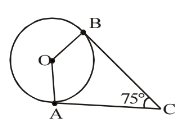Practice Test: Circles - Question 4

In figure 10.75, PA and PB are the two tangents drawn to the circle. 0 is the centre of the circle. A and B are the points of contact of the tangents PA and PB with the circle. If ∠OPA = 35°, then∠ POB =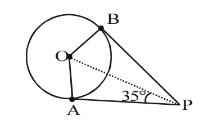Practice Test: Circles - Question 5

In fig, O is the centre of the circle. PQ is tangent to the circle and secant PAB passes through the centre O. If PQ = 5 cm and PA = 1 cm, then the radius of the circle is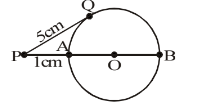Practice Test: Circles - Question 6

A tangent PQ at a point P of a circle of radius 5 cm meets a line through the centre O at a point Q such that OQ = 12 cm. Length PQ is

Practice Test: Circles - Question 7

From a point Q, the length of the tangent to a circle is 24 cm and the distance of Q from the centre is 25 cm. The radius of the circle is

Practice Test: Circles - Question 8

The length of the tangent from a point A at a circle, of radius 3 cm, is 4 cm. The distance of A from the centre of the circle is

Practice Test: Circles - Question 9

If tangents PA and PB from a point P to a circle with centre O are inclined to each other at an angle of 80° then ∠POA is equal to

Practice Test: Circles - Question 10

If TP and TQ are two tangents to a circle with centre O so that ∠POQ = 110°, then, ∠PTQ is equal to

Practice Test: Circles - Question 11

In the given figure, PT is tangent to the circle with centre O. If OT = 6 cm and OP = 10 cm then the length of tangent PT is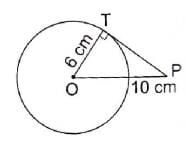Detailed Solution for Practice Test: Circles - Question 11

In right triangle PTO  By using Pythagoras theorem,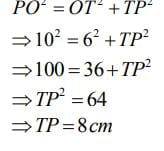PO2 = OT2 + TP

⇒ 102 = 62 + TP

⇒  TP = 100 - 36

⇒  TP = 64

⇒  TP  = 8

Practice Test: Circles - Question 12

Two circle touch each other externally at C and AB is a common tangent to the circles. Then, ∠ACB =

Detailed Solution for Practice Test: Circles - Question 12

Let P be a point on AB such that, PC is at right angles to the Line Joining the centers of the circles.

Note that, PC is a common tangent to both circles.

This is because tangent is perpendicular to radius at point of contact for any circle.

let ∠PAC= α and ∠PBC = β.

PA = PC [lengths of the tangents from an external point C]

In a triangle CAP, ∠PAC = ∠ACP = α

similarly PB = CP and ∠PCB = ∠CBP = β

now in the triangle ACB,

∠CAB + ∠CBA + ∠ACB = 180°   [sum of the interior angles in a triangle]

α + β + (α + β) = 180°   (Since ∠ACB = ∠ACP + ∠PCB = α + β.

2α + 2β = 180°

α + β = 90°

∴ ∠ACB = α + β = 90°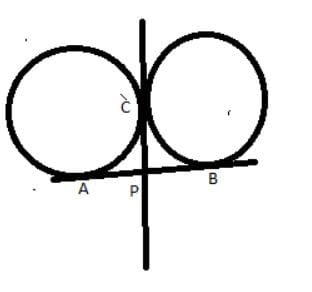Practice Test: Circles - Question 13

ABC is a right angled triangle, right angled at B such that BC = 6 cm and AB = 8 cm. A circle with centre O is inscribed in ΔABC. The radius of the circle is

Practice Test: Circles - Question 14

PQ is a tangent drawn from a point P to a circle with centre O and QOR is a diameter of the circle such that ∠POR = 120°, then ∠OPQ is

Practice Test: Circles - Question 15

If four sides of a quadrilateral ABCD are tangential to a circle, then

Practice Test: Circles - Question 16

The length of the tangent drawn from a point 8 cm away from the centre of a circle of radius 6 cm is

Detailed Solution for Practice Test: Circles - Question 16

Let P be the external point and PA and PB be the tangents and OA and OB be the radii.
So OP is the hypotenuse=8cm
Applying Pythagoras theorem,
H= P+ B2
64 = AP+ 36
AP =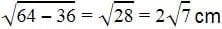Practice Test: Circles - Question 17

AB and CD are two common tangents to circles which touch each other at C. If D lies on AB such that CD = 4 cm, then AB is equal to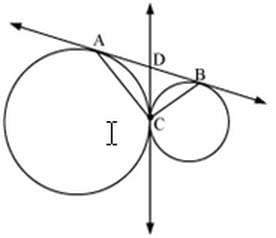Detailed Solution for Practice Test: Circles - Question 17

From the given figure, CD = 4 cm
CD=BD=4CD = BD = 4CD=BD=4 (tangents drawn from an exterior point are equal)

Practice Test: Circles - Question 18

In the adjoining figure, if AD, AE and BC are tangents to the circle at D, E and F respectively. Then,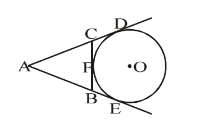Practice Test: Circles - Question 19

If the diagonals of a cyclic quadrilateral are equal, then the quadrilateral is

Detailed Solution for Practice Test: Circles - Question 19

Let ABCD be a cyclic quadrilateral having diagonals BD and AC, intersecting each other at point O.

(Consider BD as a chord)

∠BCD = 180− 90 = 90
(Considering AC as a chord)

90+ ∠ABC = 180
∠ABC = 90

Each interior angle of a cyclic quadrilateral is of 90.Hence it is a rectangle.

Practice Test: Circles - Question 20

The quadrilateral formed by angle bisectors of a cyclic quadrilateral is a:

Detailed Solution for Practice Test: Circles - Question 20

To prove: PQRS is a cyclic quadrilateral.

Proof: In △ARB, we have

1/2∠A + 1/2∠B + ∠R = 180°   ....(i)   (Since, AR, BR are bisectors of ∠A and ∠B)

In △DPC, we have

1/2∠D + 1/2∠C +  ∠P = 180°  ....(ii)   (Since, DP,CP are bisectors of ∠D and ∠C respectively)

1/2∠A + 1/2∠B + ∠R + 1/2∠D + 1/2∠C + ∠P = 180° + 180°

∠P + ∠R = 360° - 1/2(∠A  + ∠B + ∠C  + ∠D)

∠P + ∠R = 360° - 1/2 x 360° = 360° - 180°

⇒ ∠P + ∠R = 180°

As the sum of a pair of opposite angles of quadrilateral PQRS is 180°. Therefore, quadrilateral PQRS is cyclic.

Practice Test: Circles - Question 21

In the given figure, AB is the diameter of the circle. Find the value of ∠ACD :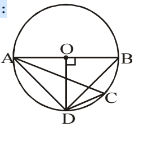Practice Test: Circles - Question 22

Find the value of ∠DCE :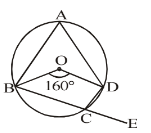Practice Test: Circles - Question 23

In the given figure, PQ is the tangent of the circle. Line segment PR intersects the circle at N and R. PQ = 15 cm, PR = 25 cm, find PN: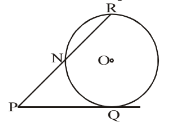Detailed Solution for Practice Test: Circles - Question 23

Given, PQ = 15 cm

PR = 25 cm

We know that, PQ² = PN × PR
15² = PN × 25
225 = PN × 25
PN = 9

Practice Test: Circles - Question 24

In the given figure, there are two circles with the centres O and O' touching each other internally at P. Tangents TQ and TP are drawn to the larger circle and tangents TP and TR are drawn to the smaller circle. Find TQ : TR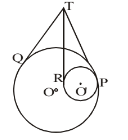Practice Test: Circles - Question 25

In the given figure, PAQ is the tangent. BC is the diameter of the circle. m ∠BAQ = 60°, find m ∠ABC :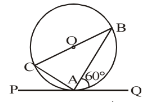Detailed Solution for Practice Test: Circles - Question 25

∠ABC = 30 degrees

## NCERT Mathematics for CAT Preparation

276 docs|149 tests
 Use Code STAYHOME200 and get INR 200 additional OFF Use Coupon Code
Information about Practice Test: Circles Page
In this test you can find the Exam questions for Practice Test: Circles solved & explained in the simplest way possible. Besides giving Questions and answers for Practice Test: Circles, EduRev gives you an ample number of Online tests for practice

## NCERT Mathematics for CAT Preparation

276 docs|149 tests